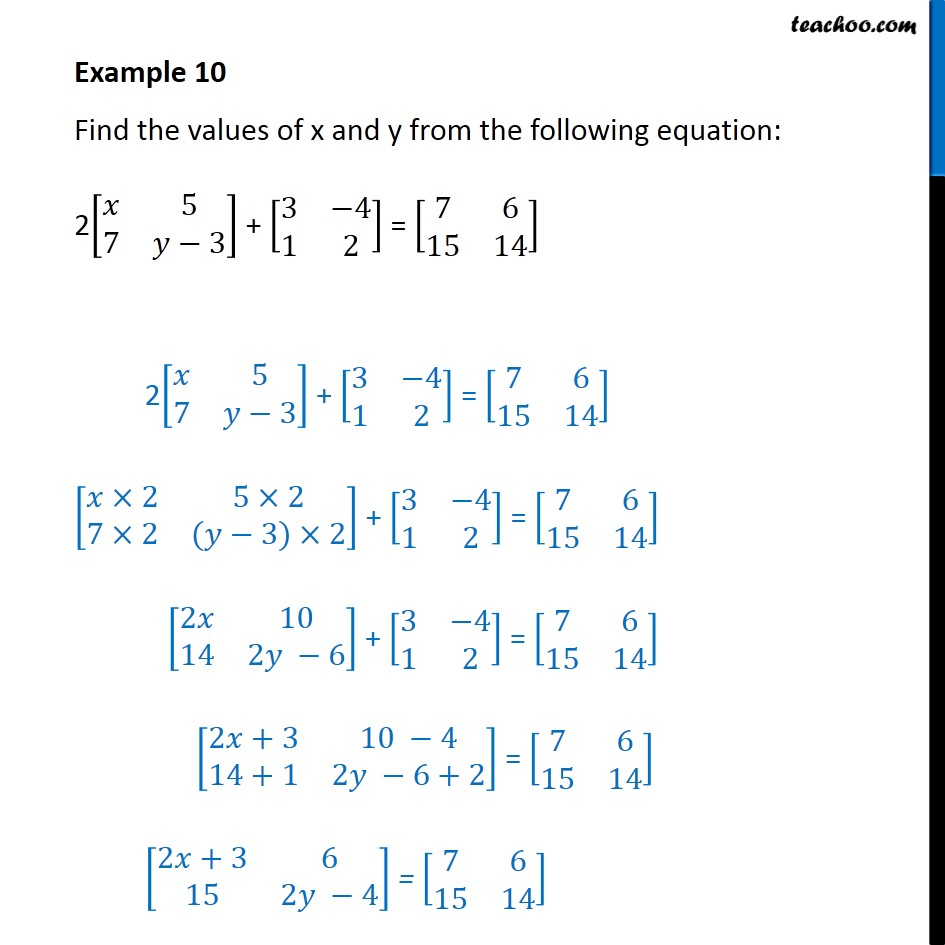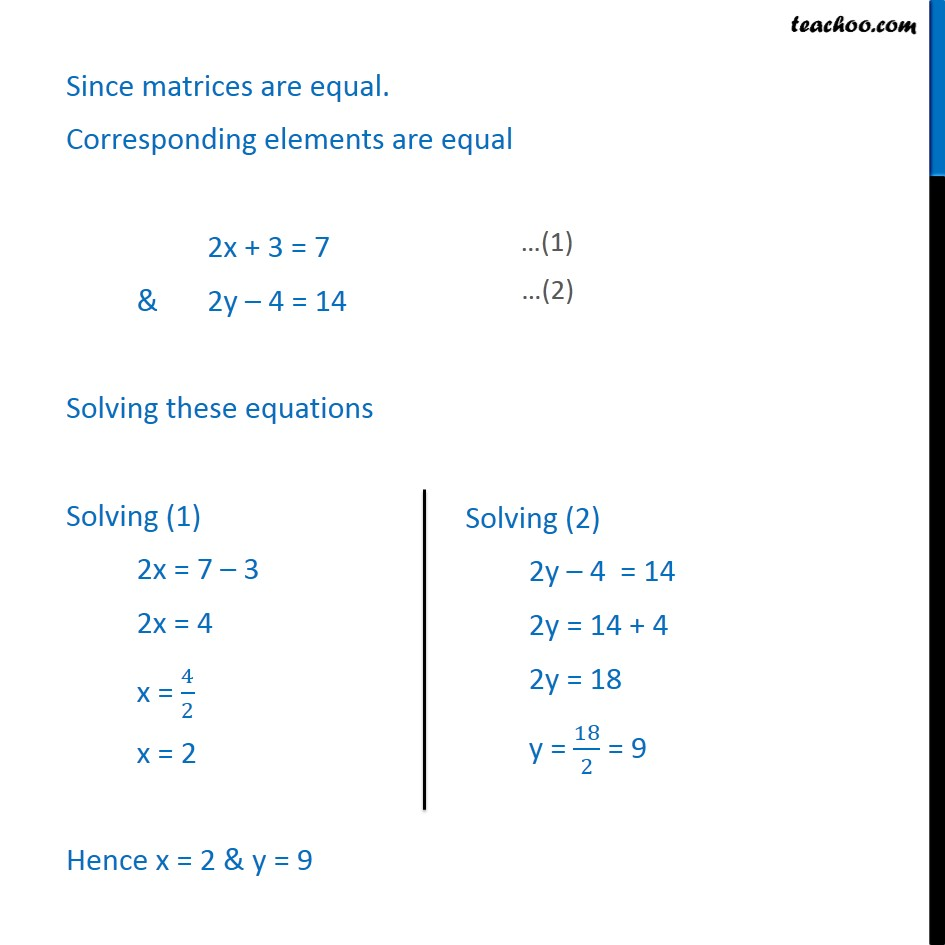Learn All Concepts of Chapter 3 Class 12 Matrices - FREE. Check - Matrices Class 12 - Full video1. Chapter 3 Class 12 Matrices
2. Serial order wise
3. Examples

Transcript

Example 10 Find the values of x and y from the following equation: 2[ 8( &5@7& 3)] + [ 8(3& 4@1&2)] = [ 8(7&6@15&14)] 2[ 8( &5@7& 3)] + [ 8(3& 4@1&2)] = [ 8(7&6@15&14)] [ 8( 2&5 2@7 2&( 3) 2)] + [ 8(3& 4@1&2)] = [ 8(7&6@15&14)] [ 8(2 &10@14&2 6)] + [ 8(3& 4@1&2)] = [ 8(7&6@15&14)] [ 8(2 +3&10 4@14+1&2 6+2)] = [ 8(7&6@15&14)] [ 8(2 +3&6@15&2 4)] = [ 8(7&6@15&14)] Since matrices are equal. Corresponding elements are equal 2x + 3 = 7 & 2y 4 = 14 Solving these equations Solving (1) 2x = 7 3 2x = 4 x = 4/2 x = 2 Hence x = 2 & y = 9

Examples

Chapter 3 Class 12 Matrices
Serial order wise